# Continuity in Calculus: Help and Review Chapter Exam

Exam Instructions:

Choose your answers to the questions and click 'Next' to see the next set of questions. You can skip questions if you would like and come back to them later with the yellow "Go To First Skipped Question" button. When you have completed the practice exam, a green submit button will appear. Click it to see your results. Good luck!

Answered 0 of 25

### Page 1

#### Question 1 1. Given y below and the intermediate value theorem, how many times will y = 0 between x = 0 and x = π?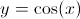#### Question 2 2. Given the function below, in what region will f(x) = 0?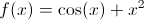#### Question 3 3. What are the regions of continuity given the following?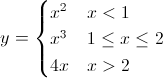#### Question 4 4. Consider the function below. According to the intermediate value theorem, is there a solution to f(x) = 0 for a value of x between -5 and 5?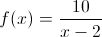#### Question 5 5. What are the regions of continuity for y defined as?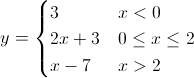### Page 3

#### Question 14 14. How many discontinuities are in this function?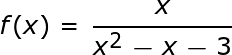#### Question 15 15. Consider the function below. According to the intermediate value theorem, is there a solution to f(x) = 4 for a value of x between 4 and 7?### Page 4

#### Question 17 17. What are the regions of continuity in this graph of f(x)?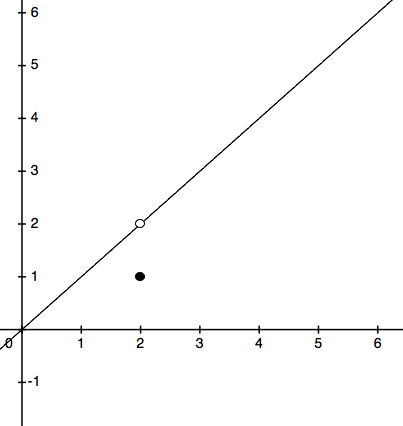#### Question 19 19. Consider the function f(x) below. Considering the intermediate value theorem and the vertical asymptote at x=0, is the following statement true or false? For any number C greater than 0, there is a positive value of x that satisfies the equation f(x)=C.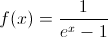#### Question 20 20. What are the regions of continuity in the graph of f(x) below?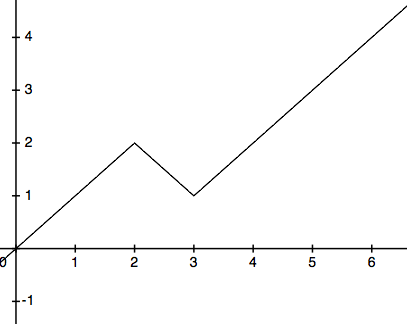### Page 5

#### Question 23 23. Which of the following continuous functions will have at least one solution to the following equation?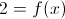#### Question 24 24. Given the values of the continuous function f(x) below, how many solutions will there be to f(x)=4.1?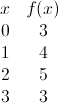#### Question 25 25. Consider the equation below. Calculate f(0), f(1) and f(2). Given that information, how many solutions are there to f(x)=-1?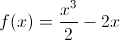#### Continuity in Calculus: Help and Review Chapter Exam Instructions

Choose your answers to the questions and click 'Next' to see the next set of questions. You can skip questions if you would like and come back to them later with the yellow "Go To First Skipped Question" button. When you have completed the practice exam, a green submit button will appear. Click it to see your results. Good luck!

Support